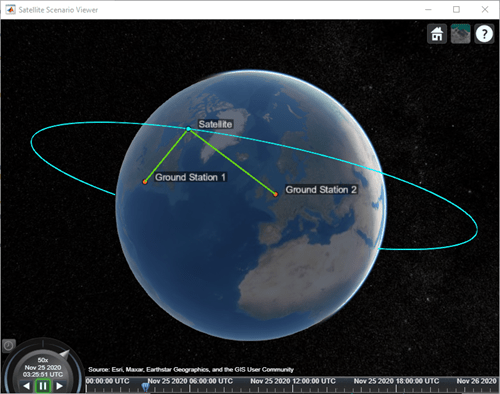Since R2021a

## Syntax

``link(asset1,asset2,...,assetN)``
``lnk = link(___,Name=Value)``
``lnk = link(___)``

## Description

example

````link(asset1,asset2,...,assetN)` adds `Link` analysis objects defined by nodes `asset1`, `asset2`, and so on.```
````lnk = link(___,Name=Value)` adds link analysis objects using additional parameters specified as name-value pairs.```
````lnk = link(___)` adds link analysis objects and returns the vector link```

## Examples

collapse all

Create a satellite scenario object.

```startTime = datetime(2020,11,25,0,0,0); stopTime = startTime + days(1); sampleTime = 60; % seconds sc = satelliteScenario(startTime,stopTime,sampleTime)```
```sc = satelliteScenario with properties: StartTime: 25-Nov-2020 StopTime: 26-Nov-2020 SampleTime: 60 AutoSimulate: 1 Satellites: [1×0 matlabshared.satellitescenario.Satellite] GroundStations: [1×0 matlabshared.satellitescenario.GroundStation] Viewers: [0×0 matlabshared.satellitescenario.Viewer] AutoShow: 1 ```

Add a satellite to the scenario.

```semiMajorAxis = 10000000; % meters eccentricity = 0; inclination = 60; % degrees rightAscensionOfAscendingNode = 0; % degrees argumentOfPeriapsis = 0; % degrees trueAnomaly = 0; % degrees sat = satellite(sc,semiMajorAxis,eccentricity,inclination,rightAscensionOfAscendingNode, ... argumentOfPeriapsis,trueAnomaly,Name="Satellite");```

Add gimbals to the satellite. These gimbals enable the satellite receiver antenna to steer to the first ground station, and its transmitter antenna to steer to the second ground station.

```gimbalrxSat = gimbal(sat); gimbaltxSat = gimbal(sat);```

```gainToNoiseTemperatureRatio = 5; % dB/K systemLoss = 3; % dB rxSat = receiver(gimbalrxSat,Name="Satellite Receiver",GainToNoiseTemperatureRatio= ... gainToNoiseTemperatureRatio,SystemLoss=systemLoss)```
```rxSat = Receiver with properties: Name: Satellite Receiver ID: 4 MountingLocation: [0; 0; 0] meters MountingAngles: [0; 0; 0] degrees Antenna: [1x1 satcom.satellitescenario.GaussianAntenna] SystemLoss: 3 decibels PreReceiverLoss: 3 decibels GainToNoiseTemperatureRatio: 5 decibels/Kelvin RequiredEbNo: 10 decibels ```

Add a transmitter to the second gimbal of the satellite.

```frequency = 27e9; % Hz power = 20; % dBW bitRate = 20; % Mbps systemLoss = 3; % dB txSat = transmitter(gimbaltxSat,Name="Satellite Transmitter",Frequency=frequency, ... power=power,BitRate=bitRate,SystemLoss=systemLoss)```
```txSat = Transmitter with properties: Name: Satellite Transmitter ID: 5 MountingLocation: [0; 0; 0] meters MountingAngles: [0; 0; 0] degrees Antenna: [1x1 satcom.satellitescenario.GaussianAntenna] SystemLoss: 3 decibels Frequency: 2.7e+10 Hertz BitRate: 20 Mbps Power: 20 decibel-watts Links: [1x0 satcom.satellitescenario.Link] ```

Specify the antenna specifications of the repeater.

```dishDiameter = 0.5; % meters apertureEfficiency = 0.5; gaussianAntenna(txSat,DishDiameter=dishDiameter,ApertureEfficiency=apertureEfficiency); gaussianAntenna(rxSat,DishDiameter=dishDiameter,ApertureEfficiency=apertureEfficiency);```

Add two ground stations to the scenario.

```gs1 = groundStation(sc,Name="Ground Station 1"); latitude = 52.2294963; % degrees longitude = 0.1487094; % degrees gs2 = groundStation(sc,latitude,longitude,Name="Ground Station 2");```

Point gimbals of the satellite towards the two ground stations for the simulation duration.

```pointAt(gimbaltxSat,gs2); pointAt(gimbalrxSat,gs1);```

Add gimbals to the ground stations. These gimbals enable the ground station antennas to steer towards the satellite.

```gimbalgs1 = gimbal(gs1); gimbalgs2 = gimbal(gs2);```

Add a transmitter to ground station `gs1`.

```frequency = 30e9; % Hz power = 40; % dBW bitRate = 20; % Mbps txGs1 = transmitter(gimbalgs1,Name="Ground Station 1 Transmitter",Frequency=frequency, ... Power=power,BitRate=bitRate);```

Add a receiver to ground station `gs2`.

```requiredEbNo = 14; % dB rxGs2 = receiver(gimbalgs2,Name="Ground Station 2 Receiver",RequiredEbNo=requiredEbNo);```

Define the antenna specifications of the ground stations.

```dishDiameter = 5; % meters gaussianAntenna(txGs1,DishDiameter=dishDiameter); gaussianAntenna(rxGs2,DishDiameter=dishDiameter);```

Point gimbals of the ground stations towards the satellite for the simulation duration.

```pointAt(gimbalgs1,sat); pointAt(gimbalgs2,sat);```

Add link analysis to transmitter `txGs1`.

`lnk = link(txGs1,rxSat,txSat,rxGs2)`
```lnk = Link with properties: Sequence: [10 4 5 11] LineWidth: 2 LineColor: [0.3922 0.8314 0.0745] ```

Determine the times when ground station `gs1` can send data to ground station `gs2` via the satellite.

`linkIntervals(lnk)`
```ans=4×8 table Source Target IntervalNumber StartTime EndTime Duration StartOrbit EndOrbit ______________________________ ___________________________ ______________ ____________________ ____________________ ________ __________ ________ "Ground Station 1 Transmitter" "Ground Station 2 Receiver" 1 25-Nov-2020 00:21:00 25-Nov-2020 00:40:00 1140 NaN NaN "Ground Station 1 Transmitter" "Ground Station 2 Receiver" 2 25-Nov-2020 03:19:00 25-Nov-2020 03:36:00 1020 NaN NaN "Ground Station 1 Transmitter" "Ground Station 2 Receiver" 3 25-Nov-2020 06:15:00 25-Nov-2020 06:36:00 1260 NaN NaN "Ground Station 1 Transmitter" "Ground Station 2 Receiver" 4 25-Nov-2020 22:20:00 25-Nov-2020 22:38:00 1080 NaN NaN ```

Visualize the link by using the Satellite Scenario Viewer.

`play(sc);`## Input Arguments

collapse all

Adds link analysis objects defined by nodes `asset1`, specified as a scalar or vector of transmitters, `asset2`, and so on, specified as a scalar or a vector of transmitters or receivers.

• If the asset in a given node is scalar, every link analysis object uses the same asset for that node position.

• If the asset in a given node is vector, the asset length must equal the number of link analysis objects.

Each link analysis object uses the corresponding element of the asset vector for that node location. The IDs of ASSET1, ASSET2, ASSET3, and so on, specify the Sequence of the link. These objects must belong to the same `satelliteScenario` object. Each link analysis object is added to the `Link` property of the corresponding transmitter in ASSET1.

### Name-Value Arguments

Specify optional pairs of arguments as `Name1=Value1,...,NameN=ValueN`, where `Name` is the argument name and `Value` is the corresponding value. Name-value arguments must appear after other arguments, but the order of the pairs does not matter.

Before R2021a, use commas to separate each name and value, and enclose `Name` in quotes.

Example: `LineWidth=2.5` sets the line width of the field of view to 2.5 pixels.

Satellite scenario viewer, specified as a scalar, vector, or array of `satelliteScenarioViewer` objects. If the `AutoSimulate` property of the scenario is `false`, adding a satellite to the scenario disables any previously available timeline and playback widgets.

## Output Arguments

collapse all

Link analysis object between input objects, returned as either a scalar or a row vector.

Note

When `AutoSimulate` of the satellite scenario is `false`, you can call `link` only when the `SimulationStatus` is `NotStarted`. Otherwise, you must call the `restart` function to erase the simulation data and reset the `SimulationStatus` to `NotStarted`.

## Version History

Introduced in R2021a